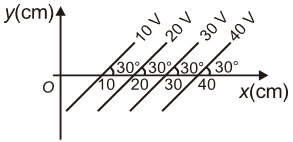You’ve reached the end of your free Videos limit.
#8 | Relation b/w Electric Field&Potential 2
(Physics) > Electrostatic Potential and Capacitance
Unable to watch the video, please try another server
Related Practice Questions :

The electric potential at a point in space due to charge Q is Q V. The value of an electric field at that point will be

1.  $\frac{\mathrm{Q}}{9}×{10}^{12}\frac{\mathrm{N}}{\mathrm{C}}$

2.  $\frac{\mathrm{Q}}{9}×{10}^{15}\frac{\mathrm{N}}{\mathrm{C}}$

3.  $\frac{\mathrm{Q}}{9}×{10}^{9}\frac{\mathrm{N}}{\mathrm{C}}$

4.

High Yielding Test Series + Question Bank - NEET 2020

Difficulty Level:

A hollow charged metal spherical shell has radius R. If the potential difference between its surface and a point at a distance 3R from the center is V, then the value of electric field intensity at a point at distance 4R from the center is

1.  $\frac{3\mathrm{V}}{19\mathrm{R}}$

2.  $\frac{\mathrm{V}}{6\mathrm{R}}$

3.  $\frac{3\mathrm{V}}{32\mathrm{R}}$

4.  $\frac{3\mathrm{V}}{16\mathrm{R}}$

High Yielding Test Series + Question Bank - NEET 2020

Difficulty Level:

The figure shows some of the equipotential surfaces. Magnitude and direction of the electric field is given by(1)  200 V/m, making an angle ${120}^{0}$ with the x-axis

(2) 100 V/m, pointing towards the negative x-axis

(3) 200 V/m, making an angle $-{600}^{0}$ with the x-axis

(4) 100 V/m, making an angle ${300}^{0}$ with the x-axis

High Yielding Test Series + Question Bank - NEET 2020

Difficulty Level:

Two spheres of radius a and b respectively are charged and joined by a wire. The ratio of electric field of the spheres is

(1) a/b

(2) b/a

(3) a2/b2

(4) b2/a2

High Yielding Test Series + Question Bank - NEET 2020

Difficulty Level:

The electric field inside a spherical shell of uniform surface charge density is -

(1) Zero

(2) Constant, less than zero

(3) Directly proportional to the distance from the centre

(4) None of the above